# NCERT Exemplar - Relations and Functions (Part - 2) Notes | EduRev

## JEE : NCERT Exemplar - Relations and Functions (Part - 2) Notes | EduRev

The document NCERT Exemplar - Relations and Functions (Part - 2) Notes | EduRev is a part of the JEE Course Mathematics (Maths) Class 12.
All you need of JEE at this link: JEE

Objective Type Questions
Q.28. Let T be the set of all triangles in the Euclidean plane, and let a relation R on T be defined as aRb if a is congruent to b ∀ a, b ∈ T. Then R is
(a) Reflexive but not transitive
(b) Transitive but not symmetric
(c) Equivalence
(d) None of these
Ans. (c)
Solution.
If a ≌ b ∀ a, b ∈ T
then a  R a ⇒ a ≌ a which is true for all a ∈ T
So, R is reflexive.
Now, aRb and bRa.
i.e., a ≌ b and b ≌ a which is true for all a, b ∈ T
So, R is symmetric.
Let aRb and bRc.
⇒ a ≌ b and b ≌ a ⇒ a ≌ c ∀ a, b, c ∈ T
So, R is transitive.
Hence, R is equivalence relation.

Q.29. Consider the non-empty set consisting of children in a family and a relation R defined as aRb if a is brother of b. Then R is
(a) Symmetric but not transitive
(b) Transitive but not symmetric
(c) Neither symmetric nor transitive
(d) Both symmetric and transitive
Ans. (b)
Solution.
Here, a  R b ⇒ a is a brother of b.
a R a ⇒ a is a brother of a which is not true.
So, R is not reflexive.
a R b ⇒ a is a brother of b.
b R a ⇒ which is not true because b may be sister of a.
⇒ a R b ≠ b R a
So, R is not symmetric.
Now, a  R b, b R c ⇒ a R c
⇒ a is the brother of b and b is the brother of c.
∴ a is also the brother of c.
So, R is transitive.

Q.30. The maximum number of equivalence relations on the set A = {1, 2, 3} are
(a) 1
(b) 2
(c) 3
(d) 5
Ans. (d)
Solution.
Here, A = {1, 2, 3} The number of equivalence relations are as follows:
R1 = {(1, 1), (1, 2), (2, 1), (2, 3), (1, 3)}
R2 = {(2, 2), (1, 3), (3, 1), (3, 2), (1, 2)}
R3 = {(3, 3), (1, 2), (2, 3), (1, 3), (3, 2)}

Q.31. If a relation R on the set {1, 2, 3} be defined by R = {(1, 2)}, then R is
(a) Reflexive
(b) Transitive
(c) Symmetric
(d) None of these
Ans. (b)
Solution:

Given that: R = {(1, 2)}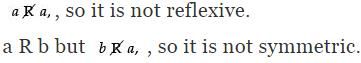a R b and b R c ⇒ a R c which is true.
So, R is transitive.

Q.32. Let us define a relation R in R as aRb if a ≥ b. Then R is
(a) An equivalence relation
(b) Reflexive, transitive but not symmetric
(c) Symmetric, transitive but not reflexive
(d) Neither transitive nor reflexive but symmetric.
Ans. (b)
Solution:

Here, aRb if a ≥ b
⇒ aRa ⇒ a ≥ a which is true, so it is reflexive.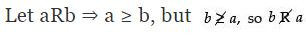R is not symmetric.
Now, a ≥ b, b ≥ c ⇒ a ≥ c which is true.
So, R is transitive.

Q.33. Let A = {1, 2, 3} and consider the relation
R = {1, 1), (2, 2), (3, 3), (1, 2), (2, 3), (1,3)}.

Then R is
(a) Reflexive but not symmetric

(b) Reflexive but not transitive
(c) Symmetric and transitive
(d) Neither symmetric nor transitive.
Ans. (a)
Solution:

Given that: R = {(1, 1), (2, 2), (3, 3), (1, 2), (2, 3), (1, 3)}
Here, 1 R 1, 2 R 2 and 3 R 3, so R is reflexive.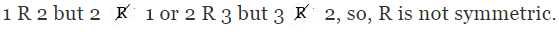1 R 1 and 1 R 2 ⇒ 1 R 3, so, R is transitive.

Q.34. The identity element for the binary operation * defined on Q ~ {0} as a * b = ab/2 ∀ a, b ∈ Q ~ {0} is
(a) 1
(b) 0
(c) 2
(d) None of these
Ans. (c)
Solution:

Given that: a * b = ab/2 ∀ a, b ∈ Q - {0}
Let e be the identity element
∴ a * e = ae/2 = a ⇒ e = 2

Q.35. If the set A contains 5 elements and the set B contains 6 elements, then the number of one-one and onto mappings from A to B is
(a) 720
(b) 120
(c) 0
(d) None of these
Ans. (c)
Solution:

If A and B sets have m and n elements respectively, then the number of one-one and onto mapping from A to B is
n! if m = n
and 0 if m ≠ n
Here, m = 5 and n = 6
5 ≠ 6
So, number of mapping = 0

Q.36. Let A = {1, 2, 3, ...n} and B = {a, b}. Then the number of surjections from A into B is
(a) nP2
(b) 2n – 2
(c) 2n – 1
(d) None of these
Ans. (d)
Solution:

Here, A = {1, 2, 3, ..., n} and B = {a, b}
Let m  be the number of elements of set A
and n  be the number of elements of set B
∴ Number of surjections from A to B is
nCm × m! as n ≥ m
Here, m = 2 (given)
∴ Number of surjections from A to B = nC2 × 2!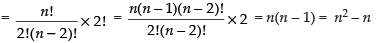Q.37. Let f: R → R be defined by f (x) = 1/x ∀ x∈ R. Then f is
(a) one-one
(b) onto
(c) bijective
(d) f is not defined
Ans. (d)
Solution:

Given that f(x) = 1/x
Put x = 0 ∴ f(x) = 1/0 = ∞
So, f(x) is not defined.

Q.38. Let f : R → R be defined by f (x) = 3x2 – 5 and g : R → R by g (x) = x/(x2 + 1). Then gof is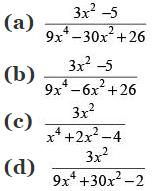Ans. (a)
Solution:

Here, f(x) = 3x2 – 5 and g(x) = x/(x2 + 1)
∴ gof = gof (x) = g[3x2 – 5]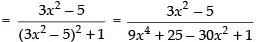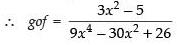Q.39. Which of the following functions from Z into Z are bijections?
(a) f(x) = x3
(b) f(x) = x + 2
(c) f(x) = 2x + 1
(d) f(x) = x2 + 1
Ans. (b)
Solution.

Given that f: Z → Z
Let x1, x2 ∈ f(x) ⇒ f(x1) = x1 + 2, f(x2) = x2 + 2
f(x1) = f(x2) ⇒ x1 + 2 = x2 + 2 ⇒ x1 = x2
So, f(x) is one-one function.
Now, let y = x + 2 ∴ x = y – 2 ∈ Z ∀ y ∈ Z
So, f(x) is onto function.
∴ f(x) is bijective function.

Q.40. Let f: R → R  be the functions defined by f (x) = x3 + 5. Then f –1 (x) is
(a) (x + 5)1/3
(b) (x – 5)1/3
(c) (5 – x)1/3
(d) 5 – x
Ans. (b)
Solution:

Given that f(x) = x3 + 5
Let y = x3 + 5 ⇒ x3 = y – 5
∴ x = (y – 5)1/3 ⇒ f – 1(x) = (x – 5)1/3

Q.41. Let f: A → B and g : B → C be the bijective functions. Then (gof)–1 is
(a) f – 1og– 1
(b) f og
(c) g – 1of – 1
(d) gof
Ans. (a)
Solution.

Here, f: A → B and g: B → C
∴  (gof)– 1 = f – 1og– 1

Q.42. Let f : R - {3/5} → R be defined by f (x) = 3x+2/5x-2. then
(a) f – 1(x) = f(x)
(b) f – 1(x) = – f(x)
(c) (fof)x = – x
(d) f-1(x) = 1/19 f(x)
Ans. (a)
Solution.

Given that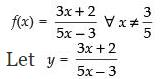⇒ y(5x – 3) = 3x + 2
⇒ 5xy – 3y = 3x + 2
⇒ 5xy – 3x = 3y + 2
⇒ x(5y – 3) = 3y + 2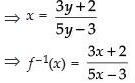⇒ f – 1(x) = f (x)

Q.43. Let f: [0, 1] → [0, 1] be defined by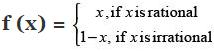Then (fof) x is
(a) Constant
(b) 1 + x
(c) x
(d) None of these
Ans. (c)
Solution.

Given that f: [0, 1] → [0, 1]
∴ f = f –1
So, (fof)x = x (identity element)

Q.44.  Let f: [2, ∞) → R be the function defined by f (x) = x2 – 4x + 5, then the range of f is
(a) R
(b) [1, ∞)

(c) [4, ∞)
(d) [5, ∞)

Ans. (b)
Solution.

Given that f(x) = x2 – 4x + 5
Let y = x2 – 4x + 5
⇒ x2 – 4x + 5 – y = 0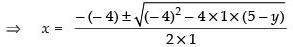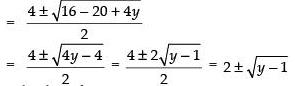∴ For real value of x, y – 1 ≥ 0 ⇒ y ≥ 1.
So, the range is [1, ∞).

Q.45. Let f: N → R be the function defined by f (x) = 2x-1/2 and g: Q → R be another function defined by g (x) = x + 2. Then (gof) 3/2 is
(a) 1
(b) – 1
(c) 7/2

(d) None of these
Ans. (d)
Solution.

Here, f(x) = 2x-1/2 and g(x) = x + 2
∴ gof(x) = g[(f(x)]
= f(x) + 2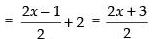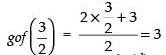Q.46. Let f: R → R be defined by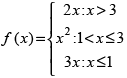Then f (– 1) + f (2) + f (4) is
(a) 9
(b) 14
(c) 5
(d) None of these
Ans. (a)
Solution.

Given that: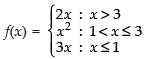∴ f(–1) + f(2) + f(4) = 3(– 1) + (2)2 + 2(4) = – 3 + 4 + 8 = 9

Q.47. Let f: R → R be given by f (x) = tan x. Then f –1 (1) is

(a) π/4
(b) {n π + π/4 : n ∈ Z}
(c) Does not exist
(d) None of these

Ans. (a)
Solution.

Given that f(x) = tan x
Let f(x) = y = tan x
⇒ x = tan– 1 y
⇒ f – 1(x) = tan– 1 (x)
⇒ f – 1(1) = tan– 1 (1)
⇒ f – 1(1) = tan 1
[tan (π/4)] = π/4

Fill in the blanks
Q.48. Let the relation R be defined in N by aRb if 2a + 3b = 30. Then R = ______.
Ans. Given that a R b : 2a + 3b = 30
⇒ 3b = 30 – 2a
⇒b = 30-2a/3
for a = 3, b = 8
a = 6, b = 6
a = 9, b = 4
a = 12, b = 2
Hence, R = {(3, 8), (6, 6), (9, 4), (12, 2)}

Q.49. Let the relation R be defined on the set
A = {1, 2, 3, 4, 5} by R = {(a, b): |a2 – b2| < 8. Then R is given by ______ .
Ans. Given that A = {1, 2, 3, 4, 5} and R = {(a, b): |a2 – b2| < 8}
So, clearly, R = {(1, 1), (1, 2), (2, 1), (2, 2), (2, 3), (3, 2), (4, 3) (3, 4), (4, 4), (5, 5)}

Q.50. Let f = {(1, 2), (3, 5), (4, 1) and g = {(2, 3), (5, 1), (1, 3)}. Then gof = ______ and fog = ______.
Ans. Here, f = {(1, 2), (3, 5), (4, 1)} and g = {(2, 3), (5, 1), (1, 3)}
gof(1) = g[f(1)] = g(2) = 3
gof(3) = g[f(3)] = g(5) = 1
gof(4) = g[f(4)] = g(1) = 3
∴ gof = {(1, 3), (3, 1), (4, 3)} fog(2) = f[g(2)] = f(3) = 5
fog(5) = f[g(5)] = f(1) = 2
fog(1) = f[g(1)] = f(3) = 5
∴ fog = {(2, 5), (5, 2), (1, 5)}

Q.51.  Let f: R → R be defined by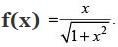Then (fofof) (x)  = ______
Ans.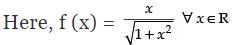fofof (x) = fof [f(x)] = f[f{f(x)}]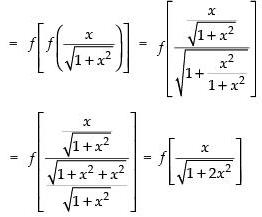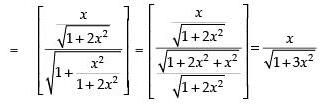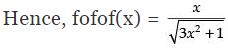Q.52. If f (x) = (4 – (x–7)3}, then f –1(x) = _______.
Ans. Given that, f(x) = [4 – (x – 7)3]
Let y = [4 – (x – 7)3]
⇒ (x – 7)3 = 4 – y
⇒ x – 7 = (4 – y)1/3 ⇒ x = 7 + (4 – y)1/3
Hence, f–1(x) = 7 + (4 – x)1/3

State True or False for the statements
Q.53. Let R = {(3, 1), (1, 3), (3, 3)} be a relation defined on the set A = {1, 2, 3}. Then R is symmetric, transitive but not reflexive.
Ans. Here, R = {(3, 1), (1, 3), (3, 3)}
(3, 3) ∈ R, so R is reflexive.
(3, 1) ∈ R and (1, 3) ∈ R, so R is symmetric.
Now, (3, 1) ∈ R and (1, 3) ∈ R but (1, 1) ∉ R
So, R is not transitive.
Hence, the statement is ‘False’.

Q.54. Let f: R → R be the function defined by f (x) = sin (3x + 2) ∀ x ∈ R. Then f is invertible.
Ans. Given that: f(x) = sin (3x + 2) ∀ x ∈ R,
f(x) is not one-one.
Hence, the statement is ‘False’.

Q.55. Every relation which is symmetric and transitive is also reflexive.
Ans. Let R be any relation defined on A = {1, 2, 3}
R = {(1, 2), (2, 1), (2, 3), (1, 3)}
Here, (1, 2) ∈ R and (2, 1) ∈ R, so R is symmetric.
(1, 2) ∈ R, (2, 3) ∈ R ⇒ (1, 3) ∈ R, so R is transitive.
But (1, 1) ∉ R, (2, 2) ∉ R and (3, 3) ∉ R.
Hence, the statement is ‘False’.

Q.56. An integer m is said to be related to another integer n if m is a integral multiple of n. This relation in Z is reflexive, symmetric and transitive.
Ans. Here, m = kn (where k is an integer)
If k = 1 m = n, so z is reflexive.
Clearly z is not symmetric but z is transitive.
Hence, the statement is ‘False’.

Q.57. Let A = {0, 1} and N be the set of natural n umbers. Then the mapping f: N → A defined by f(2n–1) = 0, f (2n) = 1, ∀ n ∈ N, is onto.
Ans. Given that A = [0, 1]
f(2n – 1) = 0 and f(2n) = 1 ∀ n ∈ N
So, f: N → A is a onto function.
Hence, the statement is ‘True’.

Q.58. The relation R on the set A = {1, 2, 3} defined as R = {{1, 1), (1, 2), (2, 1), (3, 3)} is reflexive, symmetric and transitive.
Ans. Here, R = {(1, 1), (1, 2), (2, 1), (3, 3)}
Here, (1, 1) ∈ R, so R is Reflexive.
(1, 2) ∈ R and (2, 1) ∈ R, so R is Symmetric.
(1, 2) ∈ R but (2, 3) ∉ R
So, R is not transitive.
Hence, the statement is ‘False’.

Q.59. The composition of functions is commutative.
Ans. Let f (x) = x2 and g(x) = 2x + 3
fog(x) = f[g(x)] = (2x + 3)2 = 4x2 + 9 + 12x
gof(x) = g[f(x)] = 2x2 + 3
So, fog(x) ≠ g o f(x)
Hence, the statement is ‘False’.

Q.60. The composition of functions is associative.
Ans. Let f (x) = 2x, g(x) = x – 1 and h(x) = 2x + 3
fo{goh(x)} = fo{g(2x + 3)}
= f(2x + 3 – 1) = f(2x + 2) = 2(2x + 2) = 4x + 4.
and (fog)oh(x) = (fog) {h(x)}
= fog(2x + 3)
= f(2x + 3 – 1) = f(2x + 2) = 2(2x + 2) = 4x + 4
So, fo{goh(x)} = {(fog)oh(x)} = 4x + 4
Hence, the statement is ‘True’.

Q.61. Every function is invertible.
Ans. Only bijective functions are invertible.
Hence, the statement is ‘False’.

Q.62. A binary operation on a set has always the identity element.
Ans. ‘+’ is a binary operation on the set N but it has no identity element. Hence, the statement is ‘False’.

Offer running on EduRev: Apply code STAYHOME200 to get INR 200 off on our premium plan EduRev Infinity!

## Mathematics (Maths) Class 12

209 videos|222 docs|124 tests

,

,

,

,

,

,

,

,

,

,

,

,

,

,

,

,

,

,

,

,

,

;